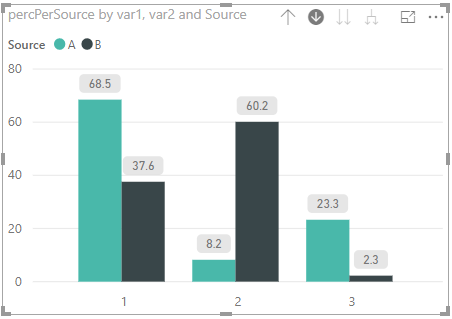4 views
in BI

## Problem

I would like to create a multi-layer histogram that shows the distribution of var1 on the first level and var2 on the second level, with a legend by source, like this:

##The value should show the percentage w.r.t. the total of a source, with all the selections and slicers applied. The percentages shown in the histogram should always sum to 100% per source.

## Example data

I have the following example data:

source  var1    var2    count

A       1       1       100

A       1       2       12

A       1       3       34

A       2       1       1612

A       2       2       23

A       2       3       43

B       1       1       200

B       1       2       320

B       1       3       12

B       2       1       1757

B       2       2       345

B       2       3       32

## What have I tried

I can achieve a total per source with the following measure without the filtering part:

percPerSource =

DIVIDE(

SUM(input[count]);

CALCULATE(

SUM(input[count]);

ALLEXCEPT(input;input[source])

)

)*100If I turn on Drill mode and click on the columns of var1 I get the following, an undesired result (the percentages do not sum to 100%):Another attempt was using the ALLSELECTED function:

percSelected =

DIVIDE(

SUM(input[count]);

CALCULATE(

SUM(input[count]);

ALLSELECTED(input[var1])

)

)*100

This shows only 100% on the var2 level:by (47.2k points)

percPerSource = DIVIDE( SUM(input[count]); CALCULATE( SUM(input[count]); FILTER( ALLSELECTED(input); input[Source] IN VALUES(input[Source]) ) ) )*100

• This takes all the selected values as the universe you are filtering on but only selects the rows that in your local filter context.• The FILTER function takes a table as its first argument and a condition as the second argument. It iterates through every row in the table passed into it and checks if the condition holds and returns a table with only the rows where the condition evaluates to True.
• The VALUES function returns a list of distinct values of the column specified evaluated within the local filter context.